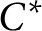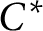Hostname: page-component-797576ffbb-bqjwj Total loading time: 0 Render date: 2023-12-02T16:38:15.527Z Has data issue: false Feature Flags: { "corePageComponentGetUserInfoFromSharedSession": true, "coreDisableEcommerce": false, "useRatesEcommerce": true } hasContentIssue false

# Ring-theoretic (In)finiteness in reduced products of Banach algebras

Published online by Cambridge University Press:  29 June 2020

## Abstract

We study ring-theoretic (in)finiteness properties—such as Dedekind-finiteness and proper infiniteness—of ultraproducts (and more generally, reduced products) of Banach algebras.

While we characterise when an ultraproduct has these ring-theoretic properties in terms of its underlying sequence of algebras, we find that, contrary to the$C^*$ -algebraic setting, it is not true in general that an ultraproduct has a ring-theoretic finiteness property if and only if “ultrafilter many” of the underlying sequence of algebras have the same property. This might appear to violate the continuous model theoretic counterpart of Łoś’s Theorem; the reason it does not is that for a general Banach algebra, the ring theoretic properties we consider cannot be verified by considering a bounded subset of the algebra of fixed bound. For Banach algebras, we construct counter-examples to show, for example, that each component Banach algebra can fail to be Dedekind-finite while the ultraproduct is Dedekind-finite, and we explain why such a counter-example is not possible for$C^*$ -algebras. Finally, the related notion of having stable rank one is also studied for ultraproducts.

## MSC classification

Type
Article
Information
Canadian Journal of Mathematics , October 2021 , pp. 1423 - 1458

## Access options

Get access to the full version of this content by using one of the access options below. (Log in options will check for institutional or personal access. Content may require purchase if you do not have access.)

## Footnotes

Horváth acknowledges with thanks the funding received from GAČR project 19-07129Y; RVO 67985840 (Czech Republic).

## References

Ben Yaacov, I., Berenstein, A., Henson, C. W., and Usvyatsov, A., Model theory for metric structures. In: Model theory with applications to algebra and analysis. Vol. 2, London Math. Soc. Lecture Note Ser., 350, Cambridge Univ. Press, Cambridge, UK, 2008, pp. 315427. http://dx.doi.org/10.1017/CBO9780511735219.011CrossRefGoogle Scholar
Blackadar, B.,$K$ -theory for operator algebras. 2nd ed., Mathematical Sciences Research Institute Publications, 5, Cambridge University Press, Cambridge, UK, 1998.Google Scholar
Dales, H. G., Banach algebras and automatic continuity. London Mathematical Society Monographs. New Series, 24, The Clarendon Press, Oxford University Press, New York, 2000.Google Scholar
Dales, H. G., Laustsen, N. J., and Read, C. J., A properly infinite Banach$\ast$ -algebra with a non-zero, bounded trace . Studia Math. 155(2003), no. 2, 107129. http://dx.doi.org/10.4064/sm155-2-2 CrossRefGoogle Scholar
Daws, M., Amenability of ultrapowers of Banach algebras . Proc. Edinb. Math. Soc. (2) 52(2009), no. 2, 307338. http://dx.doi.org/10.1017/S13091507001083 CrossRefGoogle Scholar
Dawson, T. W. and Feinstein, J. F., On the denseness of the invertible group in Banach algebras. Proc. Amer. Math. Soc. 131(2003), no. 9, 28312839. http://dx.doi.org/10.1090/S0002-9939-03-07058-8CrossRefGoogle Scholar
Draga, S. and Kania, T., When is multiplication in a Banach algebra open? Linear Algebra Appl. 538(2018), 149165. http://dx.doi.org/10.1016/j.laa.2017.10.007 CrossRefGoogle Scholar
Engelking, R., Dimension theory . Translated from the Polish and revised by the author, North-Holland Mathematical Library, 19, North-Holland Publishing Co, Amsterdam; PWN—Polish Scientific Publishers, Warsaw, 1978.Google Scholar
Farah, I., Combinatorial set theory of${C}^{\ast }$ -algebras. Springer Monographs in Mathematics, Springer, Cham, 2019. http://dx.doi.org/10.1007/978-3-030-27093-3CrossRefGoogle Scholar
Farah, I., Hart, B., Lupini, M., Robert, L., Tikuisis, A., Vignati, A., and Winter, W., Model theory of${C}^{\ast }$ -algebras. Mem. Amer. Math. Soc, to appear. arXiv:1602.08072Google Scholar
Farah, I., Hart, B., and Sherman, D., Model theory of operator algebras II: model theory. Israel J. Math. 201(2014), no. 1, 477505. http://dx.doi.org/10.1007/s11856-014-1046-7 CrossRefGoogle Scholar
Folland, G. B., A course in abstract harmonic analysis. 2nd ed., Textbooks in Mathematics, CRC Press, Boca Raton, FL, 2016.CrossRefGoogle Scholar
Friis, P. and Rørdam, M., Almost commuting self-adjoint matrices—a short proof of Huaxin Lin’s theorem. J. Reine Angew. Math. 479(1996), 121131. http://dx.doi.org/10.1515/crll.1996.479.121 CrossRefGoogle Scholar
Ghasemi, S., Reduced products of metric structures: a metric Feferman-Vaught theorem. J. Symb. Log. 81(2016), no. 3, 856875. http://dx.doi.org/10.1017/jsl.2016.20 CrossRefGoogle Scholar
Heinrich, S., Ultraproducts in Banach space theory. J. Reine Angew. Math. 313(1980), 72104. http://dx.doi.org/10.1515/crll.1980.313.72Google Scholar
Hewitt, E. and Ross, K. A., Abstract harmonic analysis. Vol. I. 2nd ed., Structure of topological groups, integration theory, group representations. Grundlehren der Mathematischen Wissenschaften, 115, Springer-Verlag, Berlin, 1979.CrossRefGoogle Scholar
Horváth, B., Banach spaces whose algebras of operators are Dedekind-finite but they do not have stable rank one. Banach Algebras and Applications, de Gruyter (editor: M. Filali), 2020, pages 165–177. http://dx.doi.org/10.1515/9783110602418 arXiv:1807.10578CrossRefGoogle Scholar
Johnson, B. E., Approximately multiplicative maps between Banach algebras. J. Lond. Math. Soc. (2) 37(1988), no. 2, 294316. http://dx.doi.org/10.1112/jlms/s2-37.2.294CrossRefGoogle Scholar
Kirchberg, E., Central sequences in${C}^{\ast }$ -algebras and strongly purely infinite algebras. In: Operator Algebras: The Abel Symposium 2004, Abel Symp., 1, Springer, Berlin, 2006, pp. 175231. http://dx.doi.org/10.1007/978-3-540-34197-0_10 CrossRefGoogle Scholar
Kochen, S., Ultraproducts in the theory of models. Ann. of Math. (2) 74(1961), 221261. http://dx.doi.org/10.2307/1970234 CrossRefGoogle Scholar
Loring, T. A., Lifting solutions to perturbing problems in${C}^{\ast }$ -algebras. Fields Institute Monographs, 8, American Mathematical Society, Providence, RI, 1997. http://dx.doi.org/10.1090/fim/008Google Scholar
Palmer, T. W., Banach algebras and the general theory of${}^{\ast }$ -algebras. Vol. I. Encyclopedia of Mathematics and its Applications, 49, Cambridge University Press, Cambridge, UK, 1994. http://dx.doi.org/10.1017/CBO9781107325777Google Scholar
Pears, A. R., Dimension theory of general spaces. Cambridge University Press, Cambridge, UK, 1975.Google Scholar
Renault, J., A groupoid approach to${C}^{\ast }$ -algebras. Lecture Notes in Mathematics, 793, Springer, Berlin, 1980.CrossRefGoogle Scholar
Rieffel, M. A., Dimension and stable rank in the$K$ -theory of${C}^{\ast }$ -algebras. Proc. Lond. Math. Soc. (3) 46(1983), no. 2, 301333. http://dx.doi.org/10.1112/plms/s3-46.2.301 CrossRefGoogle Scholar
Rørdam, M., Larsen, F., and Laustsen, N., An introduction to$K$ -theory for${C}^{\ast }$ -algebras. London Mathematical Society Student Texts, 49, Cambridge University Press, Cambridge, UK, 2000.Google Scholar
Takesaki, M., Theory of operator algebras. III. Encyclopaedia of Mathematical Sciences, 127, Operator Algebras and Non-commutative Geometry, 8, Springer-Verlag, Berlin, 2003. http://dx.doi.org/10.1007/978-3-662-10453-8Google Scholar
Zemánek, J., Idempotents in Banach algebras. Bull. London Math. Soc. 11(1979), 177183. http://dx.doi.org/10.1112/blms/11.2.177 CrossRefGoogle Scholar